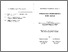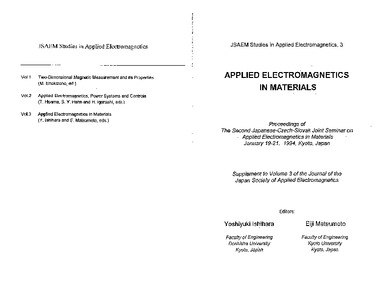# On the Current Input in 3-D Finite Element Analysis using Tetrahedron Edge Finite Element

Cingoski, Vlatko and Kaneda, Kazufumi and Yamashita, Hideo (1995) On the Current Input in 3-D Finite Element Analysis using Tetrahedron Edge Finite Element. Applied electromagnetic in materials, Proceedings of The Second Japanese-Czech-Slovak Seminar, 3. pp. 357-367.Preview Text applied 7.pdf Download (1MB) | Preview

## Abstract

This paper considers with one of the main problems in the recently developed edge finite element method: inputting the source current. Source current must be input in such a manner to satisfy the solenoidal condition for each finite element, a condition essential in developing a numerically stable system of algebraical equations. Satisfaction of the solenoidal source current condition is especially difficult in cases where the shape of the soils is either complicated of symmetrical along axis, which necessitates the use of tetrahedral edge elements. Using electric vector potential as an auxiliary function instead of using current density vectors directly, in this paper the authors propose a simple and efficient algorithm vectors directly, in this paper the authors propose a simple model. Obtained results together with conclusions are also presented.

Item Type: Article Engineering and Technology > Electrical engineering, electronic engineering, information engineering Faculty of Electrical Engineering Vlatko Cingoski 18 Dec 2012 08:45 30 Sep 2013 10:04 https://eprints.ugd.edu.mk/id/eprint/3919View Item